﻿ Introduction to Newton Fractals – Alice Ryhl

# Introduction to Newton Fractals

When I try to learn a new programming language, I don't write a hello world. Instead, I write a generator of newton fractals. Here's an example of a newton fractal: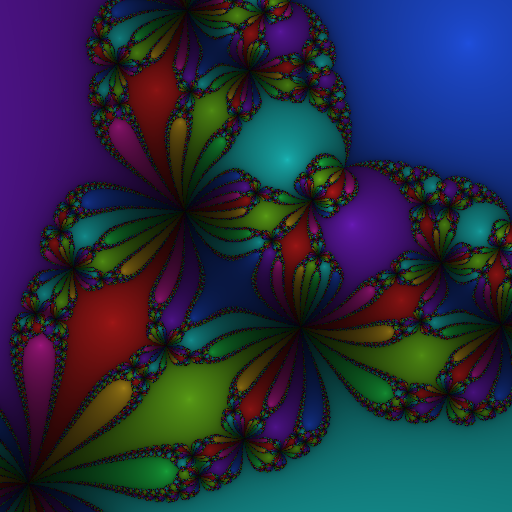Newton fractal for z8+15z416 in the square from 0.5+0.5i to 1.5+1.5i.

## What is a newton fractal?

To understand what newton fractals are, you first need to understand what Newton's method is.

Newton's method is a way to approximate solutions to an equation of the form f(x) = 0. To use the method, first try to guess a solution x0 to the equation, and use the following formula:

The value x1 that you calculate with this formula will be closer to a solution than x0 was. If you want an even better approximation to the solution, just repeat the formula on x1 to obtain x2. You can repeat this as many times as you want, to obtain a solution that is as precise as you want.

Some equations have multiple solutions, and the solution you converge to is determined by the initial guess. This is what forms the basis for creating the fractal. The wikipedia page says the method works on real-valued functions, but it works just fine on complex-valued functions as well.

An interesting thing to investigate is then, which root do the different starting guesses converge to? Below is shown some F# code which creates an image with each pixel given a colour corresponding to the root that it converged to. I picked the function f(x) = x3−1 as the one used in the iteration step, since it's a rather simple function, but not so simple it doesn't show any fractal behaviour.

``````open System
open System.Drawing

(* pick the region of the complex plane to show *)
let width = 512
let height = 512
let center = new C(0.0, 0.0)
let scale = 256.0 (* how many pixels per unit *)

(* pick a complex number from the pixel coordinate *)
let tc x w = (float x - 0.5*float w) / scale
let transform (x,y) = center + new C(tc x width, tc y height)

(* how many times we improve the guess *)
let iterations = 100

(* the function and its derivative *)
let f x = x*x*x - new C(1.0, 0.0)
let fd x = new C(3.0, 0.0) * x*x

(* computes one iteration *)
let step z = z - (f z) / (fd z)

(* computes n iterations *)
let rec compute_pixel z n = if n = 0 then z else compute_pixel (step z) (n - 1)

(* picks a color for the pixel
* this function uses the function hsltorgb,
* which is not included here for briefness *)
let complex_to_color (z:C) =
let (r,g,b) = hsltorgb (z.Phase, 0.75, 0.5)
Color.FromArgb(r, g, b)

(* functions to compute the color of a pixel *)
let newton z = compute_pixel z iterations
let newton_pixel x y = transform (x,y) |> newton |> complex_to_color

(* create an image *)
let bitmap = new Bitmap(width, height)
for px = 0 to width-1 do
for py = 0 to height-1 do
bitmap.SetPixel(px, py, newton_pixel px py)
bitmap.Save("fractal.png", System.Drawing.Imaging.ImageFormat.Png)
bitmap.Dispose
``````

The code shown here also depends on the function `hsltorgb` which is excluded for brevity, and is available here. Running this code produces the following image: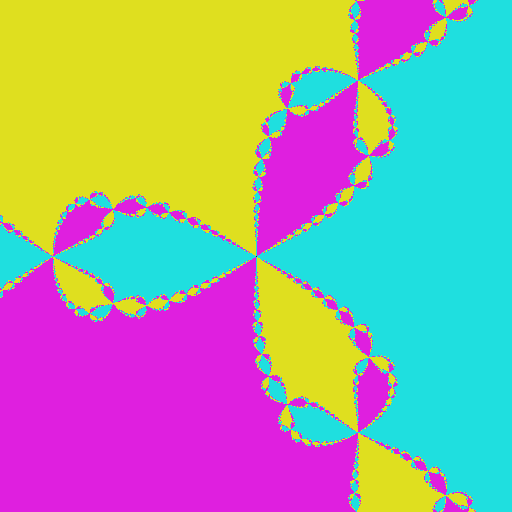Newton fractal for z31 in the square from −1−i to 1+i.

With a preliminary result like this, it's clear that this has the potential to create very interesting and pretty images.

## Prettier fractals

In the image above I ran 100 iterations for every pixel, but this is probably way more than necessary. Instead, we can stop when the iteration changes the result by something very small. This also allows us to create a more interesting image: we can change the darkness depending on the amount of iterations we made before stopping. We make the following chances to the code:

``````(* now only performs as many iterations as necessary,
* returns the amount of iterations performed too *)
let compute_pixel (z:C) =
let rec aux z n =
let z2 = step z
if (z2 - z).Magnitude < 0.001 || n > 100 then (z2,n) else aux z2 (n+1)
aux z 0

(* incorporate the amount of iterations in the color *)
let complex_to_color (z:C,n) =
let (r,g,b) = hsltorgb (z.Phase, 0.75, 0.5 / (1.+0.18*float n))
Color.FromArgb(r, g, b)

let newton z = compute_pixel z
``````

Running the updated code produces the following image: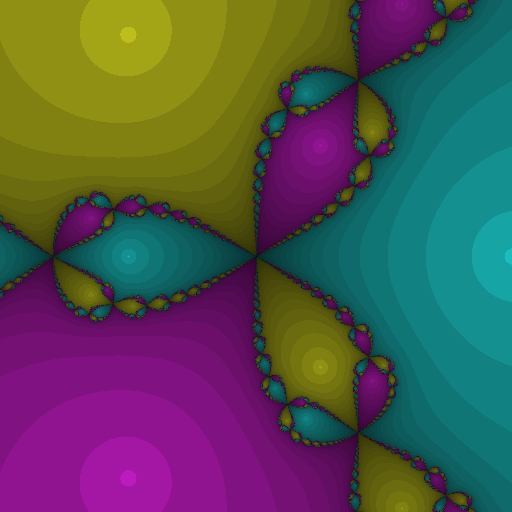Newton fractal for z31 in the square from −1−i to 1+i.

It would be even nicer if we could make the brightness change smoothly. If you're okay with foregoing the optimization of only running as many iterations as necessary, you can make the colour change smoothly. In the previous image the darkness was chosen from the amount of iterations performed. To have the darkness change smoothly, compute the sum of the distances from the values xi to the root it converged to, and base the darkness on that sum. The following changes to the code incorporates this:

``````let compute_pixel (z:C) =
let rec aux z n =
let z2 = step z
if n > 100 then (z2,0.0) else
let (zf,sum) = aux z2 (n+1)
let delta = (z - zf).Magnitude
(zf, sum + delta)
aux z 0

(* incorporate the amount of iterations in the color *)
let complex_to_color (z:C,n) =
let (r,g,b) = hsltorgb (z.Phase, 0.75, 0.5 / (1.+0.18*n))
Color.FromArgb(r, g, b)
``````

Running the updated code produces the following image: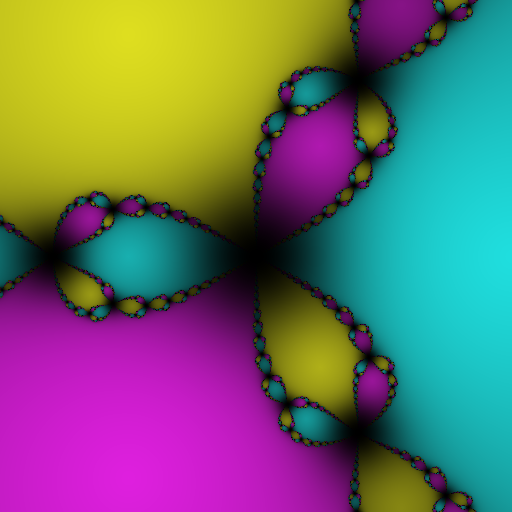Newton fractal for z31 in the square from −1−i to 1+i.

This is better than before, but adding the distances linearly doesn't quite give the gradient I want. The following code applies a sublinear function to the distance before adding them together:

``````let compute_pixel (z:C) =
t rec aux z n =
let z2 = step z
if n > 100 then (z2,0.0) else
let (zf,sum) = aux z2 (n+1)
let delta = (z - zf).Magnitude
(zf, sum + 1.0 - 1./(1.+exp (delta+3.5)-exp 3.5))
x z 0
``````

Running the updated code produces the following image: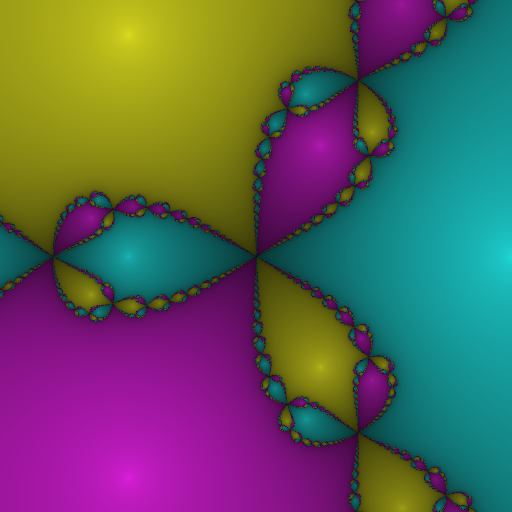Newton fractal for z31 in the square from −1−i to 1+i.

The final code is available here.